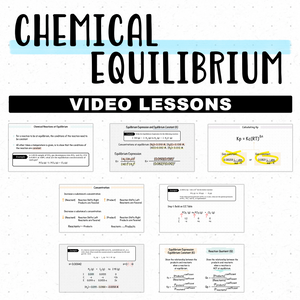10% OFF 2 NOTES, 20% OFF 4 NOTES, 30% OFF 6 NOTES# The Complete Course on Chemical Equilibrium

Regular price
\$50.00
Sale price
\$40.00

This course covers:

• The Equilibrium Definition and Concept
• How to write equilibrium expressions, K, Kc, Kp
• How to Build an Ice Table
• When to use or not to use an ICE Table
• When to use or not to use the quadratic formula
• Finding Kp and Kc
• Finding the equilibrium concentrations
• Finding the equilibrium partial pressures
• Finding Kp using the Kp=Kc(RT)^∆n formula
• Finding Kc using the Kp=Kc(RT)^∆n formula
• Using the 5% rule or x is small assumption
• What to do when there are two positive x values
• The difference between Q and K
• Examples on predicting the direction of the reaction
• An example that requires the use of the ideal gas law (PV=nRT)
• Le Chatelier's Principle
• Trick Questions